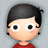#### 学习笔记TF007:Tensor、Graph、Op、Variable、占位符、Session、名称作用域、Board综合例子

[None]代表任意长度向量，[]代表标量。update环节更新各Variable对象以及将数据传入TensorBoard汇总Op。与交换工作流分开，独立名称作用域包含Variable对象，存储输出累加和，记录数据流图运行次数。独立名称作用域包含TensorBoard汇总数据，tf.scalar_summary Op。汇总数据在Variable对象更新完成后才添加。

``````import tensorflow as tf#导入TensorFlow库
#构建数据流图
graph = tf.Graph()#显式创建Graph对象
with graph.as_default():#设为默认Graph对象
with tf.name_scope("variables"):#创建Variable对象名称作用域
global_step = tf.Variable(0, dtype=tf.int32, trainable=False, name="global_step")#记录数据流图运行次数的Variable对象，初值为0，数据类型为32位整型，不可自动修改，以global_step标识
total_output = tf.Variable(0.0, dtype=tf.float32, trainable=False, name="total_output")#追踪模型所有输出累加和的Variable对象，初值为0.0，数据类型为32位浮点型，不可自动修改，以total_output标识
with tf.name_scope("transformation"):#创建变换计算Op名称作用域
with tf.name_scope("input"):#创建独立输入层名称作用域
a = tf.placeholder(tf.float32, shape=[None], name="input_placeholder_a")#创建占位符，接收一个32位浮点型任意长度的向量作为输入，以input_placeholder_a标识
with tf.name_scope("intermediate_layer"):#创建独立中间层名称作用域
b = tf.reduce_prod(a, name="product_b")#创建创建归约乘积Op，接收张量输入，输出张量所有分量(元素)的乘积，以product_b标识
c = tf.reduce_sum(a, name="sum_c")#创建创建归约求和Op，接收张量输入，输出张量所有分量(元素)的求和，以sum_c标识
with tf.name_scope("output"):#创建独立输出层名称作用域
with tf.name_scope("update"):
with tf.name_scope("summaries"):#创建数据汇总Op名称作用域
avg = tf.div(update_total, tf.cast(increment_step, tf.float32), name="average")#计算平均值，输出累加和除以数据流图运行次数，把运行次数数据类型转换为32位浮点型，以average标识
tf.summary.scalar(b'output_summary',output)#创建输出节点标量数据统计汇总，以output_summary标识
tf.summary.scalar(b'total_summary',update_total)#创建输出累加求和标量数据统计汇总，以total_summary标识
tf.summary.scalar(b'average_summary',avg)#创建平均值标量数据统计汇总，以average_summary标识
with tf.name_scope("global_ops"):#创建全局Operation(Op)名称作用域
init = tf.global_variables_initializer()#创建初始化所有Variable对象的Op
merged_summaries = tf.summary.merge_all()#创建合并所有汇总数据的Op
#运行数据流图
sess = tf.Session(graph=graph)#用显式创建Graph对象启动Session会话对象
writer = tf.summary.FileWriter('./improved_graph', graph)#启动FileWriter对象，保存汇总数据
sess.run(init)#运行Variable对象初始化Op
def run_graph(input_tensor):#定义数据注图运行辅助函数
"""
辅助函数：用给定的输入张量运行数据流图，
并保存汇总数据
"""
feed_dict = {a: input_tensor}#创建feed_dict参数字典，以input_tensor替换a句柄的tf.placeholder节点值
_, step, summary = sess.run([output, increment_step, merged_summaries], feed_dict=feed_dict)#使用feed_dict运行output不关心存储，运行increment_step保存到step，运行merged_summaries Op保存到summary
#用不同的输入用例运行数据流图
run_graph([2,8])
run_graph([3,1,3,3])
run_graph()
run_graph([1,2,3])
run_graph([11,4])
run_graph([4,1])
run_graph([7,3,1])
run_graph([6,3])
run_graph([0,2])
run_graph([4,5,6])
writer.flush()#将汇总数据写入磁盘
writer.close()#关闭FileWriter对象，释放资源
sess.close()#关闭Session对象，释放资源
``````WX20170513-142835#2x.png" />